Programmable shading - idea by Rob Cook. "Connect the blocks" to hook up shader pieces into complex functionality.

Pat Hanrahan formalized the idea into RSL.

* you'd write them "blind", ie. not knowing when on a surface it gets called

* RenderMan will provide detailed neighborhood info. for current shading point

* you'd write the shader in ASCII (.sl), compile it, generate a more efficient machine-readable version (.slo)

* the shader (.slo file) will be referenced in the RIB file containing the surface that we wish to shade

* shaders can be thought of as external (to RIB) shading "plugins" which RenderMan uses during rendering
• surface
• displacement
• light
• atmosphere (volume)
• imager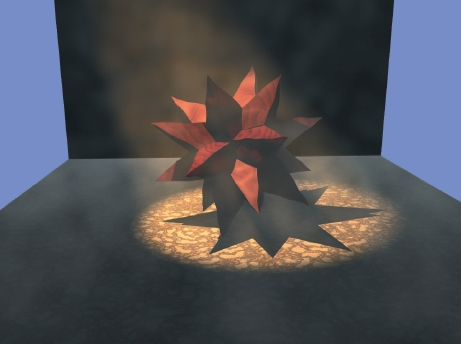```surface shaderskel()
{
```
```surface constant()
{
Oi = Os;
Ci = Os * Cs;
}
```
What are Oi, Os, Ci and Cs? They are "magic" (pre-defined) variables..
A shader has three sources of data: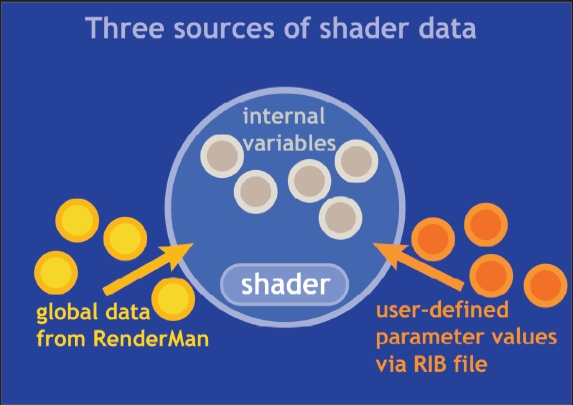```surface matte( float Ka=1, Kd=1 )
{
normal Nf; // 'local' variable, normal 'type'

Nf = faceforward(normalize(N),I);

Oi = Os;
Ci = Os * Cs * ( Ka*ambient() + Kd*diffuse(Nf) ) ;
}
```
Variables are of specific types in RSL. Available types are float, color, point, vector, normal, matrix, string.

ambient() and diffuse() are 'built-in functions'. RSL has a rich, expressive set of such built-ins.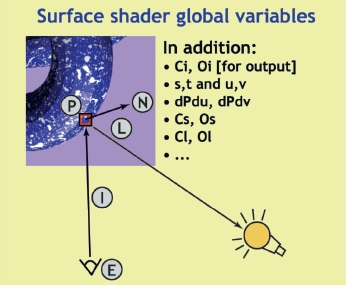Here are the main variables available to you inside a shader:

• P - shading point location
• N - shading point normal
• I - camera view direction
• E - camera location
• L - light direction
• Cs - inherent surface color
• Os - inherent surface opacity
• s,t - texture coords of shading point

• Ci - outgoing shading point color
• Oi - outgoing shading point opacity
• Cl - outgoing light color [valid only in 'light' shaders]
For a complete list of globals, see the Spec.
```surface metal (float Ka=1, Ks=1, roughness=.1)
{
normal Nf;
vector V;

Nf = faceforward(normalize(N), I) ;
V = normalize(-I) ;

Oi = Os;
Ci = Os * Cs * ( Ka*ambient() + Ks*specular(Nf,V,roughness) );
}
```
Note that incoming parameters ("arguments") have (need) default values. Also, note that there is no formal return value! All output occurs through pre-determined globals (eg. Ci, Oi..).
In addition to built-in functions, you can write your own, and call them (make use of them) repeatedly in your shader. Functions let you isolate, package, share/reuse shader blocks.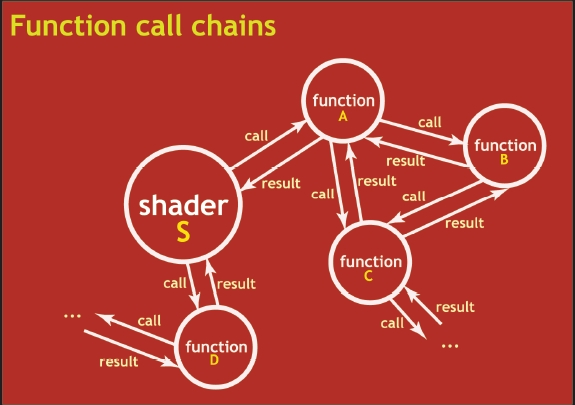Our next shader uses noise() calls to create a turbulence pattern.

```// not used, is here just for studying - see turbul() below which *is* used
float turb(varying point PP)
{
varying point PPP = PP/2;	/* frequency adjustment (S-shaped curve) */
float pixelsize = sqrt(area(PPP));
float twice = 2 * pixelsize;
float scale, weight, turbulence;

/* compute turbulence */
turbulence = 0;
for (scale = 1; scale > twice; scale /= 2) {
turbulence += scale * abs(noise(PPP/scale)-0.5);
}
/* gradually fade out of highest freq component near visibility limit */
if (scale > pixelsize) {
weight = (scale / pixelsize) - 1;
weight = clamp(weight, 0, 1);
turbulence += weight * scale * abs(noise(PPP/scale)-0.5);
}

float ct = turbulence;
// float ct = clamp(turbulence,0.75,1);
// ct -= 0.75;
// ct *= 4.0;

// printf("Turb: %f\n",ct);

return ct;

}

// this simpler version is used instead of turb() above
float turbul(varying point PP;float n)
{
float t;
if(n==4)
t = 0.5333*(noise(PP) + 0.5*(noise(2*PP)) + 0.25*(noise(4*PP)) + 0.125*(noise(8*PP)));
else if (n==3)
t = 0.5714*(noise(PP) + 0.5*(noise(2*PP)) + 0.25*(noise(4*PP)));
else if (n==2)
t = 0.6667*(noise(PP) + 0.5*(noise(2*PP)));
else if (n==1)
t = noise(PP);

return t;
}

surface smarble(float Ka=1, Kd=.6, Ks=0.4, roughness=.2,Ksin=1.0,freq=0.1,n=4;)
{
varying point PP;
varying float cmi;
varying normal Nf;
varying vector V;
color diffusecolor ;

Nf = faceforward( normalize(N), I);
V = -normalize(I);

// float t = 0.5*(1+sin(Ksin*3.1415*turbul(PP,n)));
float u = turbul(PP,n);
// print(u);

color base = color spline(u,
color (0.8, 0.2, 0.05),
color (0.8, 0.2, 0.05),
color (0.8,0.5,0.3),
color (0.6,0.594,0.58),
color (0.3,0.3,0.4),
color (0.05, 0.05, 0.1),
color (0.8,0.79,0.77),
color (0.8,0.8,0.79)
);

Ci = Os*Cs*  ((base*(Ka*ambient() + Kd*diffuse(Nf) + Ks * specular(Nf,V,roughness)))) ;

}// smarble()

// Surface "smarble" "freq" 1.5 "Kd" .46 "Ks" 2.5 "Ka" .4 "roughness" .2

```

Here is a RIB file with an 'smarble' call. Note that the 'n' parameter in the shader is what specifies how many octaves of frequencies to add (n can be ints between 1 through 4). For n=1,2,3,4 (specified via the RIB file) we get the following renders. You can see how by layering add'l higher frequencies (with corresponding lower amplitudes) we evolve pure noise into turbulence.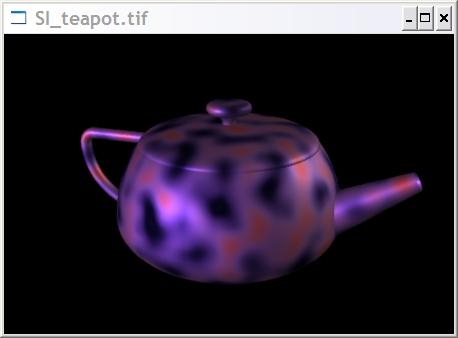n=1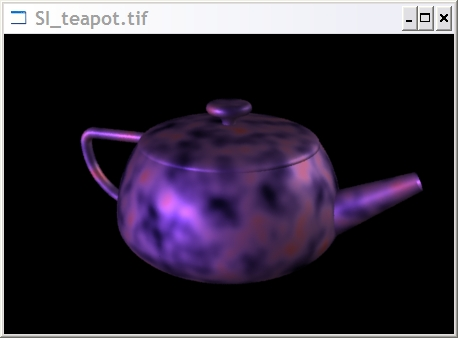n=2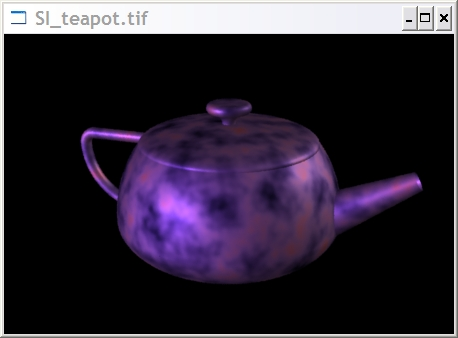n=3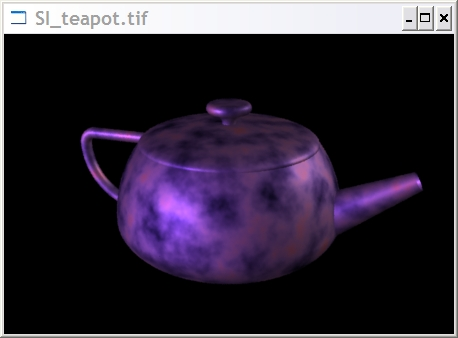n=4animated GIF version of the above four images

```surface showN()
{
point PP;
normal Nf;
vector V;
color Ct, Ot;

normal NN = normalize(N);
vector posNorm = 0.5*(vector(1,1,1)+NN);
color posCol = color(comp(posNorm,0),comp(posNorm,1),comp(posNorm,2));

Oi = Os;
Ci = Oi*posCol;
}

```
This shader reads a texture map (a specially processed image file) and uses the map colors to set Ci.
```surface tex(string tmap="generic.tex";)
{

if(tmap!="")
{
float alpha;
Ci = color texture(tmap,s,t);

/* alpha = texture(tmap,s,t);
Ci = alpha*Ci; */
}

Oi = Os;
Ci *= (Cs*Oi);

}// tex()
```
Compile the above into tex.slo, then use this RIB file to render it. You will also need a hues.tex texture map, which you would have to create starting with hues.tif (a standard TIFF image file), using the PRMan command 'txmake', like so:
```txmake hues.tif hues.tex
```
The hues.tif image looks like this: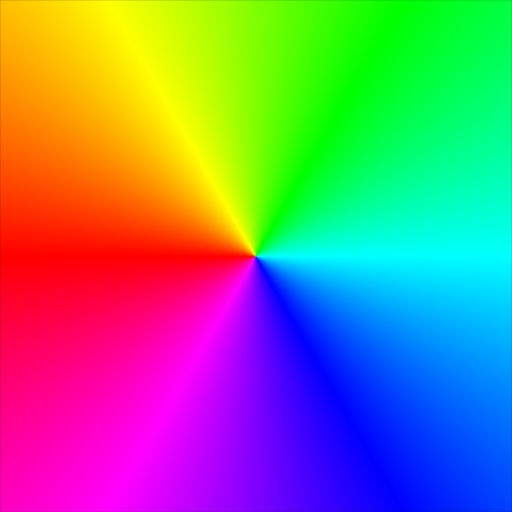Our rendered surface that uses the above texture map (via our 'tex' shader shown earlier) looks like this: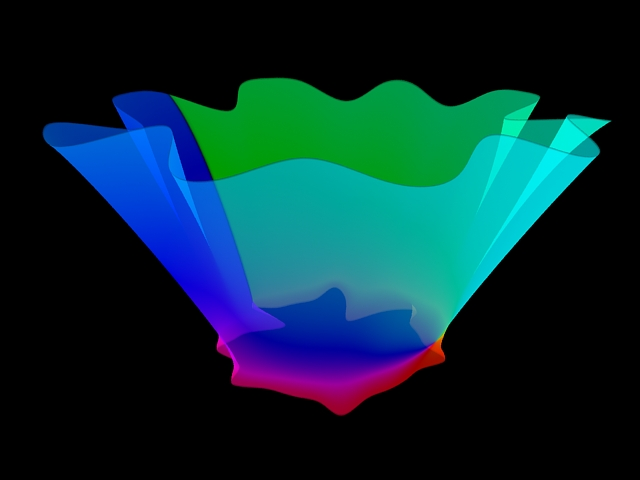RSL lets you "write your own lights".
```light
noisypointlt(
float  intensity=1 ;
color  lightcolor=1 ;
point from = point "shader" (0,0,0) ;
float freq=1.0, mixf=0.5;
)
{
illuminate(from)
{
float n = (noise(Ls*freq)); //
Cl = (mixf*n+intensity)*lightcolor;
}
}

Sample RIB to test our 'noisypointlt' light:

# teapot.rib
# Author: Scott Iverson
# Date: 6/7/95
#
Display "TeapotAfter.tif" "framebuffer" "rgb"
Format 900 600 1

Projection "perspective" "fov" 30

Translate 0 0 25

Rotate -22 1 0 0
Rotate 19 0 1 0

Translate 0 -3 0

WorldBegin

LightSource "ambientlight" 1 "intensity" .26
LightSource "distantlight" 2 "intensity" .26 "from" [-4 6 -7] "to" [0 0 0]  "lightcolor" [1.0 0.4 1.0]
LightSource "distantlight" 3 "intensity" .36 "from" [14 6 7] "to" [0 -2 0] "lightcolor" [0.0 1.0 1.0]
LightSource "noisypointlt" 4 "intensity" 0 "from" [0 10 0] "freq" 1

Surface "plastic"

Color [1 .6 1]

# spout

AttributeBegin
Sides 2
Translate 3 1.3 0
Rotate 30 0 0 1
Rotate 90 0 1 0
Hyperboloid  1.2 0 0 .4 0 5.7  360
AttributeEnd

# handle

AttributeBegin
Translate -4.3 4.2 0
TransformBegin
Rotate 180 0 0 1
Torus 2.9 .26 0 360 90
TransformEnd
TransformBegin
Translate -2.38 0 0
Rotate 90 0 0 1
Torus 0.52 .26 0 360 90
TransformEnd
Translate -2.38 0.52 0
Rotate 90 0 1 0
Cylinder .26 0 3.3 360
AttributeEnd

# body

AttributeBegin
Rotate -90 1 0 0
TransformBegin
Translate 0 0 1.7
Scale 1 1 1.05468457
Sphere 5 0 3.12897569 360
TransformEnd
TransformBegin
Translate 0 0 1.7
Scale 1 1 0.463713017
Sphere 5 -3.66606055 0 360
TransformEnd
AttributeEnd

# top

AttributeBegin
Rotate -90 1 0 0
Translate 0 0 5
AttributeBegin
Scale 1 1 0.2051282
Sphere 3.9 0 3.9 360
AttributeEnd

Translate 0 0 .8
AttributeBegin
Orientation "rh"
Sides 2
Torus 0.75 0.45 90 180 360
AttributeEnd
Translate 0 0 0.675
Torus 0.75 0.225 -90 90 360

Disk 0.225 0.75 360
AttributeEnd

WorldEnd

```